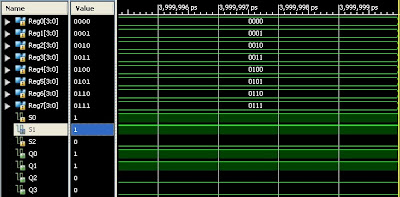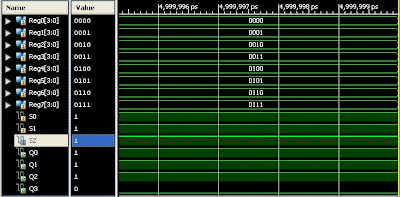## Wednesday, April 24, 2013

### How to Design Multiplexer 8 Input to 4 Output using Xilinx ISE Design Suite

Robometricschool. - In microcontroller project edition, we want to share with you how to make design Microprocessor that very important in microcontroller part. As we know that Microprocessor consist of three main parts likes Arithmetic Logic Unit (ALU), Register Unit (RU),and Control Unit (CU). All of the three parts can be designed using basic digital gate.

One of the part to make Microprocessor unit is Multiplexer. In this project we will show you how to design multiplexer 8 input (R0....R7) to 4 output (Q0...Q4) using Xilinx ISE Design Suite. Main reason why we design this schematic diagram because we will design Microprocessor 4 bits only. In here we will show you schematic diagram of Multiplexer 8 input to 4 output, show you the result of the simulation of this schematic diagram.

To make you easy follow this lesson, you can take free download tutorial e-book of using Xilinx ISE Design Suite from this blog.

Multiplexer Performance

As we know that main work of Multiplexer is open data from input to output according select data that used as like table 1 below:Table 1. Truth Table of Multiplexer 8 input to 4 output

From table 1 above we can describe that with adjust S0, S1, and S2 we can get output according data R0 until R7 from input. As example when we adjust S0=0, S1=0, and S2=0 we can get output data from data R0.

Schematic Diagram of Multiplexer 8 Input to 4 OutputFigure 1. Schematic Diagram of Multiplexer 8 Input to 4  Output

Convert to Component Symbol

After we drawing schematic diagram like in figure 1 above, we can continue to make component symbol of multiplexer 8 input to 4 output using Xilinx ISE Design Suite to make easy when we need use this schematic in other design. The component symbol of this schematic like in figure 2 below:Figure 2. Symbol of Multiplexer 8 input to 4 output

Multiplexer 8 Input to 4 Output Simulation Result

Using Xilinx ISE Design Suite software we can know performance of Multiplexer 8 input to 4 output, shall it suitable with truth table of this schematic or no, if it is suitable, it indicate that our design is true. Here are simulation result of multiplexer 8 input to 4 output that we get.Figure 3. Simulation result S0=1, S1 and S2 =0Figure 4. Simulation result S0 and S1=1, S2 =0Figure 5. Simulation result S0, S1, S3=1

According the simulation result our multiplexer 8 input to 4 output can be concluded that our design can work properly like truth table 1.

Reference:

#### Post a Comment

Thank's for your visiting and reading this article, please share your idea with comment here